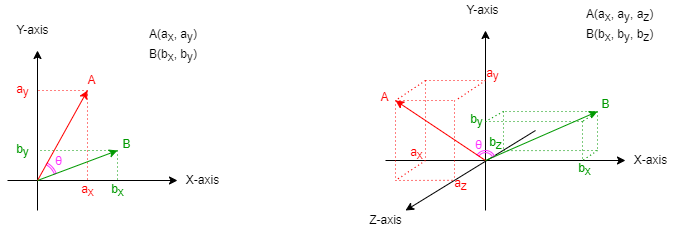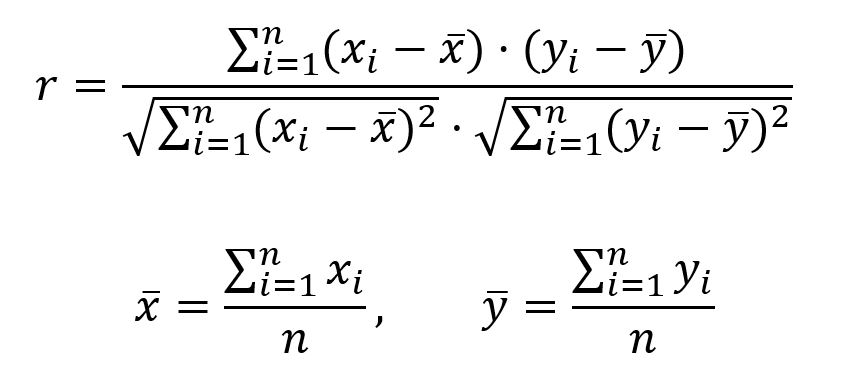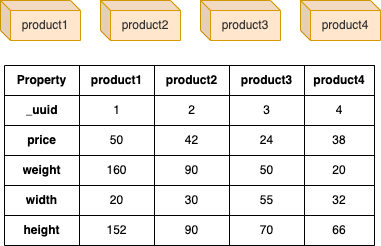# Change Nickname

Current Nickname:
Search
v4.0
v4.0

# Pearson Correlation Coefficient

## Overview

The Pearson correlation coefficient measures the linear correlation between two variables. The Pearson correlation coefficient between two nodes in graph is calculated by using N properties of node to form two N-dimensional vectors.

## Basic Concept

### Vector

Vector is one of the basic concepts in Advanced Mathematics, vectors in low dimensional spaces are relatively easy to understand and express. The following diagram shows the relationship between vectors A, B and coordinate axes in 2- and 3-dimensional spaces respectively, as well as the angle `θ` between them:When comparing two nodes in graph, N properties of node are used to form the two N-dimensional vectors.

### Pearson Correlation Coefficient

The range of Pearson correlation coefficient values is [-1,1]; let `r` to denote the Pearson correlation coefficient, then:

• `r > 0` indicates positive correlation, i.e. as one variable becomes larger, the other variable becomes larger;
• `r < 0` indicates negative correlation, i.e. as one variable becomes larger, the other variable becomes smaller;
• `r = 1` or `r = -1` indicates that two variables can be described by a linear equation, i.e. them fall on the same line;
• `r = 0` indicates that there is no linear correlation (but may exist some other correlations).

Pearson correlation coefficient is defined as the quotient of the covariance and standard deviation between two variables, and is calculated as:## Special Case

### Lonely Node, Disconnected Graph

Theoretically, the calculation of Pearson Correlation Coefficient between two nodes does not depend on the existence of edges in the graph. Regardless of whether the two nodes to be calculated are lonely nodes or whether they are in the same connected component, it does not affect the calculation of their Pearson Correlation Coefficient.

### Self-loop Edge

The calculation of Pearson Correlation Coefficient has nothing to do with edges.

### Directed Edge

The calculation of Pearson Correlation Coefficient has nothing to do with edges.

## Results and Statistics

The graph below has 4 product nodes (edges are ignored), use properties price, weight, weight and height to form vector:Algorithm results: Calculate Pearson Correlation Coefficient between product1 and other 3 products, return `node1`, `node2` and `similarity`

node1 node2 similarity
1 2 0.9987851216012547
1 3 0.4743838031328631
1 4 0.21049415016958328

Algorithm statistics: N/A

## Command and Configuration

• Command: `algo(similarity)`
• Configurations for the parameter `params()`:
Name Type
Default
Specification
Description
ids / uuids []`_id` / []`_uuid` / Mandatory IDs or UUIDs of the first set of nodes to be calculated, only need to configure one of them
ids2 / uuids2 []`_id` / []`_uuid` / Mandatory IDs or UUIDs of the second set of nodes to be calculated, only need to configure one of them
node_schema_property []`@<schema>?.<property>` / Numeric node property, LTE needed; at least two properties are required Node properties to form the dimensions of the vector
type string cosine jaccard / overlap / cosine / pearson / euclideanDistance / euclidean Measurement of the similarity; jaccard means to calculate Jaccard similarity, overlap means to calcualte overlap similarity, cosine means to calcualte cosine similarity, pearson means to calculate Pearson correlation coefficient, euclideanDistance means to calculate Euclidean distance, euclidean means to calcualte normalzied Euclidean distance
limit int -1 >=-1 Number of node pairs `uuids` × `uuids2` to return; return all results if sets to -1 or not set

Example: Calculate Pearson Correlation Coefficient of nodes UUID = 1,2 and nodes UUID = 3,4 through properties price and weight

``````algo(similarity).params({
uuids: [1,2],
uuids2: [3,4],
node_schema_property: [price, weight],
type: "pearson"
}) as p
return p
``````

## Algorithm Execution

#### 1. File Writeback

Configuration Data in Each Row
filename `node1`,`node2`,`similarity`

Example: Calculate Pearson Correlation Coefficient between node UUID = 1 and other nodes through properties price, weight, width and height, write the algorithm results back to file named pearson

``````algo(similarity).params({
uuids: ,
uuids2: [2,3,4],
node_schema_property: [price,weight,width,height],
type: "pearson"
}).write({
file:{
filename: "pearson"
}
})
``````

#### 2. Property Writeback

Not supported by this algorithm.

#### 3. Statistics Writeback

This algorithm has no statistics.

### Direct Return

Alias Ordinal Type
Description
Column Name
0 []perNodePair Node pair and its similarity `node1`, `node2`, `similarity`

Example: Calculate Pearson Correlation Coefficient between node UUID = 1 and other nodes through properties price, weight, width and height, define algorithm results as alias named similarity and return the results

``````algo(similarity).params({
uuids: ,
uuids2: [2,3,4],
node_schema_property: [price,weight,width,height],
type: "pearson"
}) as similarity
return similarity
``````

### Streaming Return

Alias Ordinal Type
Description
Column Name
0 []perNodePair Node pair and its similarity `node1`, `node2`, `similarity`

Example: Calculate Pearson Correlation Coefficient between node UUID = 1 and other nodes through properties price, weight, width and height, define algorithm results as alias named similarity, return 2 results

``````algo(similarity).params({
uuids: ,
uuids2: [2,3,4],
node_schema_property: [price,weight,width,height],
type: "pearson"
}).stream() as similarity
return similarity limit 2
``````

### Real-time Statistics

This algorithm has no statistics.